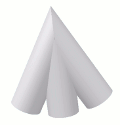# Wye Jointback to other joints

A Wye Joint is the intersection of the ends of three pipes to create a "Y" shape  (also know as a bifurcation).

Two templates are generated, a trunk template for the main trunk pipe and a branch template that is to be used twice, once for each branch, as both branches are cut identically.

Note that because one of the branch pipes is flipped over to form the joint, the triangle and diamond alignment marks will also need to be swapped when aligning that second branch.

## Parameter Constraints:

Joint Name:  name of this joint which is unique to this project or currently opened joints

Pipe OD: (Outside Diameter) must be a positive number

Pipe Wall: (Wall Thickness) must be a positive number, less than half Pipe OD

Wye Angle: must be a number between 0 deg. and 180 deg. This angle is half the angle between the two legs of the Wye.  Another way to look at it is that this angle is the amount that the legs are shifted out of alignment with the trunk pipe.

## Creative Uses:

 This may not look like a Wye but this type of structural joint is possible with a Wye Angle greater than 90 deg. Wye Angle is 150 deg.A "T" joint can be made with three pipes coming together at the joint. Wye Angle is 90 deg.Three pipes joined with equal spacing. If you want four equally spaced pipes, use the Cross Joint. For five or more equally spaced pipes, set Wye Angle to 180-(360/n) where n is the number of pipes.  Then use the trunk template on all n pipes. Wye Angle is 60 deg.